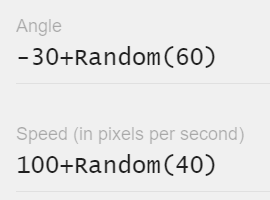# Is my interpretation of Random function correct?Just want to ask the meaning for -30+Random(60) is it the angle will be a random number from -30 to 30

and

100+Random (40) will be a random number from 100 to140?

Thanks!

hi
random(x) is a number between 0 and x-1, so for your second option you will have numbers between 100 and 139.
There is the function Randominrange(x,y)
generates numbers between x and y
The same applies for -30+random (60)

Thanks @xisco. Do know you know the range of

0.3+Random(5)/10? I saw this in on of the example but cant make out the range and dont understand why not just include the maximum number inside (), why need to separately divide?

I assume Random() only deals with integers.
0.3+Random(5)/10 = 0.3 + 0.0-0.5 = 0.3-0.8

1 Like